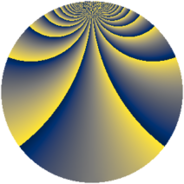# Properties

 Label 1183.2.bjLevel $1183$ Weight $2$ Character orbit 1183.bj Rep. character $\chi_{1183}(9,\cdot)$ Character field $\Q(\zeta_{39})$ Dimension $2856$ Sturm bound $242$

# Related objects

## Defining parameters

 Level: $$N$$ $$=$$ $$1183 = 7 \cdot 13^{2}$$ Weight: $$k$$ $$=$$ $$2$$ Character orbit: $$[\chi]$$ $$=$$ 1183.bj (of order $$39$$ and degree $$24$$) Character conductor: $$\operatorname{cond}(\chi)$$ $$=$$ $$1183$$ Character field: $$\Q(\zeta_{39})$$ Sturm bound: $$242$$

## Dimensions

The following table gives the dimensions of various subspaces of $$M_{2}(1183, [\chi])$$.

Total New Old
Modular forms 2952 2952 0
Cusp forms 2856 2856 0
Eisenstein series 96 96 0

## Trace form

 $$2856q - 14q^{2} - 5q^{3} + 102q^{4} - 11q^{5} - 46q^{6} - 30q^{7} - 40q^{8} - 243q^{9} + O(q^{10})$$ $$2856q - 14q^{2} - 5q^{3} + 102q^{4} - 11q^{5} - 46q^{6} - 30q^{7} - 40q^{8} - 243q^{9} - 11q^{10} + q^{11} - 15q^{12} - 48q^{13} - 26q^{14} - 7q^{15} + 90q^{16} - 22q^{17} - 10q^{18} - 26q^{19} - 54q^{20} - 32q^{21} - 26q^{22} + 16q^{23} - 53q^{24} + 98q^{25} - 13q^{26} - 14q^{27} - 18q^{28} - 48q^{29} + 59q^{30} - 26q^{31} - 81q^{32} + q^{33} - 80q^{34} - 31q^{35} + 65q^{36} + 2q^{37} - 373q^{38} + 56q^{39} - 17q^{40} - 41q^{41} + 100q^{42} - 46q^{43} - 57q^{44} + 12q^{45} + 23q^{46} - 11q^{47} - 50q^{48} + 44q^{49} - 54q^{50} + 3q^{51} + 19q^{52} - 220q^{53} - 82q^{54} - 74q^{55} - 25q^{56} - 139q^{57} - 147q^{58} - 134q^{59} - 24q^{60} - q^{61} - 56q^{62} - 158q^{63} - 260q^{64} - 53q^{65} + 46q^{66} + 231q^{67} - 111q^{68} - 118q^{69} - 102q^{70} + 33q^{71} + 73q^{72} + 4q^{73} - 216q^{74} - 447q^{75} - 172q^{76} - 36q^{77} - 83q^{78} - 25q^{79} - 34q^{80} - 251q^{81} - 17q^{82} + 2q^{83} + 213q^{84} + 47q^{85} + 143q^{86} - 34q^{87} + 85q^{88} + 15q^{89} - 60q^{90} + 35q^{91} - 154q^{92} + 115q^{93} + q^{94} - 216q^{95} - 47q^{96} - 100q^{97} - 75q^{98} - 48q^{99} + O(q^{100})$$

## Decomposition of $$S_{2}^{\mathrm{new}}(1183, [\chi])$$ into newform subspaces

The newforms in this space have not yet been added to the LMFDB.